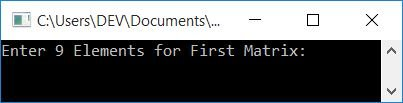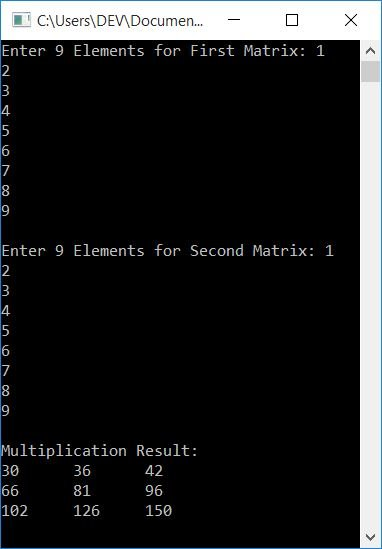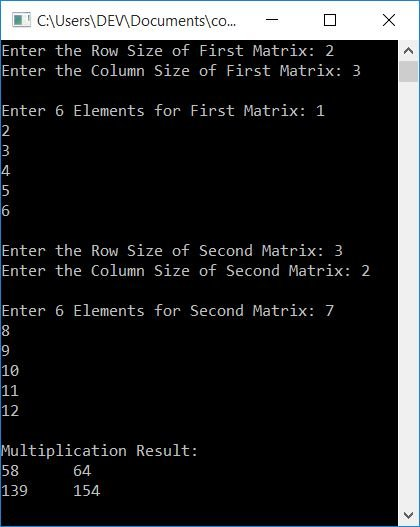# C++ Program to Multiply Two Matrices

In this article, you will learn and get code on matrix multiplication in C++. Here is the list of programs on matrix multiplication available in this article:

Before going through these programs, if you're not aware of the steps used to multiply two matrices, refer to matrix multiplication steps to get all the information you need about matrix multiplication.

## Matrix Multiplication in C++

To multiply two matrices in C++ programming, you have to ask the user to enter elements for both the first and second matrix. Now apply the formula to multiply two matrices and initialize the multiplication result's element to the third matrix one by one as shown in the program given below.

This program does not allow the user to enter the matrix size; instead, it requests 9 elements for the first matrix and 9 elements for the second 3*3 matrix. Later on, the same program that allows you to enter the size of the matrix is given.

The question is, "Write a program in C++ to multiply two matrices." Here is its answer:

```#include<iostream>
using namespace std;
int main()
{
int matOne, matTwo, matThree;
int i, j, k, sum=0;
cout<<"Enter 9 Elements for First Matrix: ";
for(i=0; i<3; i++)
{
for(j=0; j<3; j++)
cin>>matOne[i][j];
}
cout<<"\nEnter 9 Elements for Second Matrix: ";
for(i=0; i<3; i++)
{
for(j=0; j<3; j++)
cin>>matTwo[i][j];
}
// Multiplying two matrices...
for(i=0; i<3; i++)
{
for(j=0; j<3; j++)
{
sum=0;
for(k=0; k<3; k++)
sum = sum + (matOne[i][k] * matTwo[k][j]);
matThree[i][j] = sum;
}
}
cout<<"\nMultiplication Result:\n";
for(i=0; i<3; i++)
{
for(j=0; j<3; j++)
cout<<matThree[i][j]<<"\t";
cout<<endl;
}
cout<<endl;
return 0;
}```

This Program was built and runs under the Code::Blocks IDE. Here is its sample run:Now supply or enter nine numbers for the first matrix's nine elements, and nine numbers for the second matrix's nine elements. And then press the ENTER key to multiply both the matrices and print the third matrix (which  stores the multiplication result of the two given matrices), as shown in the snapshot given below:### Multiply Two Matrices of a Given Size in C++

This program multiplies two matrices of a given size that the user specifies at run-time. That is, in addition to asking to enter elements for both matrices, this program first receives the size and then asks to enter elements of the given size for both the first and second matrix.

```#include<iostream>
using namespace std;
int main()
{
int matOne, matTwo, matThree;
int matOneRow, matOneCol, matTwoRow, matTwoCol;
int i, j, k, sum;
cout<<"Enter the Row Size of First Matrix: ";
cin>>matOneRow;
cout<<"Enter the Column Size of First Matrix: ";
cin>>matOneCol;
cout<<"\nEnter "<<matOneRow*matOneCol<<" Elements for First Matrix: ";
for(i=0; i<matOneRow; i++)
{
for(j=0; j<matOneCol; j++)
cin>>matOne[i][j];
}
cout<<"\nEnter the Row Size of Second Matrix: ";
cin>>matTwoRow;
cout<<"Enter the Column Size of Second Matrix: ";
cin>>matTwoCol;
cout<<"\nEnter "<<matTwoRow*matTwoCol<<" Elements for Second Matrix: ";
for(i=0; i<matTwoRow; i++)
{
for(j=0; j<matTwoCol; j++)
cin>>matTwo[i][j];
}
if(matOneCol != matTwoRow)
{
cout<<"\nMultiplication Not Possible!\n";
return 0;
}
// Multiplying the two matrix...
for(i=0; i<matOneRow; i++)
{
for(j=0; j<matTwoCol; j++)
{
sum = 0;
for(k=0; k<matOneCol; k++)
sum = sum + (matOne[i][k] * matTwo[k][j]);
matThree[i][j] = sum;
}
}
cout<<"\nMultiplication Result:\n";
for(i=0; i<matOneRow; i++)
{
for(j=0; j<matTwoCol; j++)
cout<<matThree[i][j]<<"\t";
cout<<endl;
}
cout<<endl;
return 0;
}```

Here is its sample run with the following user inputs:

• 2 as the size of the row for the first matrix
• 3 as the size of the column for the first matrix
• 1, 2, 3, 4, 5, 6 as six elements for the first matrix
• 3 as the size of the row for the second matrix
• 2 as the column size for the second matrix
• 7, 8, 9, 10, 11, 12 as six elements for the second matrix

After providing these inputs, press the ENTER key to multiply the two given matrices and print the multiplication result as shown in the sample output given below:Note: To define matrix multiplication, the number of columns in the first matrix must be equal to the number of rows in the second matrix.

Therefore, before starting the multiplication, we checked whether the value of matOneCol was equal to the value of matTwoRow or not. If the condition is met, matrix multiplication cannot be defined; otherwise, define matrix multiplication and print the result as shown in the preceding program.

#### The same program in different languages

C++ Quiz

« Previous Program Next Program »